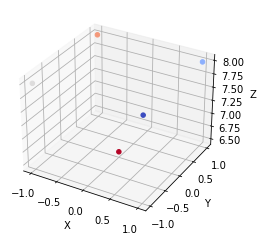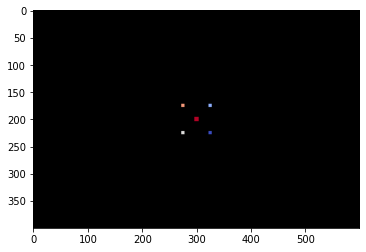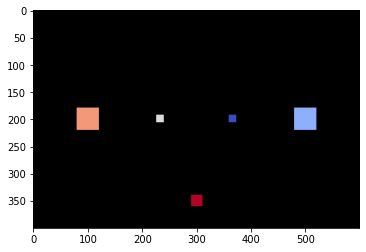In questo notebook, a partire da alcuni punti in 2D visti da una telecamera, si devono calcolare le coordinate dei punti nel piano immagine di una seconda telecamera disposta in alto rispetto alla prima e rivolta a 90° verso il basso.

In :
import numpy as np
import matplotlib.pyplot as plt
from matplotlib import cm
from PIL import Image, ImageDraw
from ipynb.fs.full.cv_functions import h
In :
# define the initial 2D points and their depths
p0 = np.array([[ 1., 1.,-1.,-1., 0.],
[-1., 1.,-1., 1., 0.],
[ 1., 1., 1., 1., 1.]])
d0 = np.array([ 8., 8., 8., 8., 6.5])
print(p0)
print(d0)
[[ 1.  1. -1. -1.  0.]
[-1.  1. -1.  1.  0.]
[ 1.  1.  1.  1.  1.]]
[8.  8.  8.  8.  6.5]
In :
# 3D points:
P0 = np.insert(p0.copy(), 2, d0, 0)
print(P0)
# plot the points in 3D
fig = plt.figure()
c = np.linspace(0,1,num=P0.shape)
ax.set_xlabel("X")
ax.set_ylabel("Y")
ax.set_zlabel("Z")
[[ 1.   1.  -1.  -1.   0. ]
[-1.   1.  -1.   1.   0. ]
[ 8.   8.   8.   8.   6.5]
[ 1.   1.   1.   1.   1. ]]
Out:
<mpl_toolkits.mplot3d.art3d.Path3DCollection at 0x7f23c9563198>Projection on a pinhole camera plane¶

The intrinsics matrix is given. The central projection point (the origin of the coordinate frames) is behind the image plane, but the image y axis is reversed (downwards) as is typical with computer images. For this reason the camera intrinsics on the y value have a minus sign.

Note that the points in the following cell are represented in homogenous coordinates, i.e. the 3D points P0 have the X, Y, Z and "1" components. In this form they cannot be directly multiplied with K matrix. For this reason, an initial projection via a 3x4 projection matrix (e.g. np.eye(3,4)) is performed.

In :
# intrinsics camera matrix: f=1cm, scale=200px/cm, sensor resolution 600x400
f = 1
K = np.array([
[f*200, 0, 300],
[0, -f*200, 200],
[0,  0, 1]
])
Kpj = K@np.eye(3,4)
# Project points on the first camera and show them
p0s = (Kpj@P0)
p0sh= (p0s/p0s[2,:]).astype(int)
p0sh
Out:
array([[325, 325, 275, 275, 300],
[225, 175, 225, 175, 200],
[  1,   1,   1,   1,   1]])
In :
image = Image.new('RGB', (600,400))
draw = ImageDraw.Draw(image)
for i in range(p0sh.shape):
Z = p0s[2,i]
size = 20*f/Z
color = tuple(map(lambda x: int(x*255), cm.get_cmap('coolwarm')(c[i])[:3]))
draw.rectangle([p0sh[0,i]-size, p0sh[1,i]-size, p0sh[0,i]+size,
p0sh[1,i]+size], fill=color, outline=color)
plt.imshow(image)
Out:
<matplotlib.image.AxesImage at 0x7f23c9482828>A) Compute the rigid transform between the two camera views: the second view is closer to the points and rotated -pi/2 rad around the x axis in order to have a view from upwards. The translation is a closeup (along Y after the rotation) and a movement upwards (along Z after the rotation).

B) Then project the points on the second camera plane and drw them, as before.

In :
Tst = np.eye(4)
# Compute Tst
In :
# Project on the second camera
# ...
P1 = # ...
p1 = # ...
print('Points in the initial reference:\n', P0)
print('Points transformed:\n', P1)
print('Points transformed and projected (non-homogeneous):\n', p1)
p1h = (p1/p1[2,:]).astype(int)
print('Points projected in the new reference frame:\n', p1h)
Points in the initial reference:
[[ 1.   1.  -1.  -1.   0. ]
[-1.   1.  -1.   1.  -0. ]
[ 8.   8.   8.   8.   6.5]
[ 1.   1.   1.   1.   1. ]]
Points transformed:
[[ 1.   1.  -1.  -1.   0. ]
[ 0.   0.   0.   0.  -1.5]
[ 3.   1.   3.   1.   2. ]
[ 1.   1.   1.   1.   1. ]]
Points transformed and projected (non-homogeneous):
[[1100.  500.  700.  100.  600.]
[ 600.  200.  600.  200.  700.]
[   3.    1.    3.    1.    2.]]
Points projected in the new reference frame:
[[366 499 233 100 300]
[199 199 199 199 349]
[  1   1   1   1   1]]

Visual hint: With this transformation two of the points lie on the transformed image plane (Orange and Light blue). The four point that compose the ideal basis of the pyramid are all at X=0.

In :
image2 = Image.new('RGB', (600,400))
# Draw image